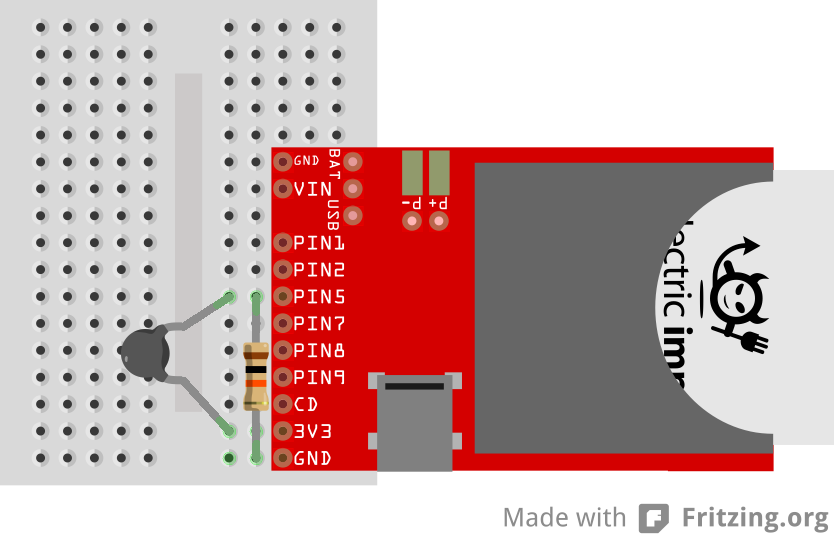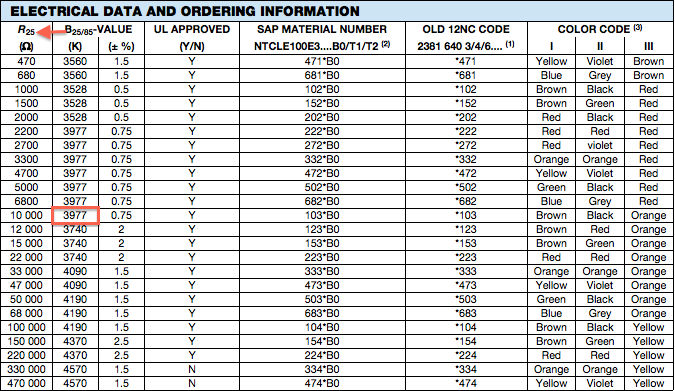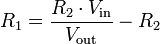This example will guide you through the process of connecting a thermistor to your imp, and polling its value at a specified interval.

This example assumes you have a basic familiarity with Electric Imp’s impCentral™. We suggest working through our Getting Started Guide before trying this example.

### What you will need

Note The following instructions were written with the imp001 in mind, but they can be readily adapted to any other imp and breakout board.

For this example you are going to need the following:

• 1 x April board
• 1 x imp001 card
• 1 x 10KΩ NTC thermistor
• 1 x 10kΩ resistor

### Circuit#### Hook Up Instructions

1. Connect one of the thermistor to 3V3 on the imp.
2. Connect the other end of the thermistor to **PIN5* on the imp.
3. Connect one of the 10kΩ resistor to PIN5 on the imp.
4. Connect the other end of the 10kΩ resistor to GND.

### Code

In impCentral, create a new Product called “Examples” (if you have not tried another of these basic hardware examples) and a new Development Device Group called “thermistor”. Assign your device to the new Device Group, then copy and paste the following code into the code editor’s ‘Device Code’ pane and hit ‘Build and Force Restart’.

#### What’s going on

The resistance of a thermistor chances based on how hot or cold the component is. Using this knowledge, we can build a voltage divider circuit, and do a bit of math to determine what the current temperature is.

Before we start, we’re going to need two values from the thermistor’s datasheet. This example uses a 10kΩ NTCLE100E3 thermistor:• B-Value3977
• Nominal Temperature (t0) 25 Celsius = 298.15 Kelvin

Once we have these values, we’re ready to configure our pin, and set some constants:

``````therm <- hardware.pin5;
therm.configure(ANALOG_IN);

const B_THERM = 3977.0;
const T0_THERM = 298.15;
const R2 = 10000.0;
``````

We can use these values to calculate the current temperature based on the resistance of the thermistor. Since we’ve built a voltage divider circuit, we know that:Since all of the values except for R2 are know, we can easily solve this equation:

• R2 = 10,000Ω (the resistance of the bottom-side resistor)
• Vin = 3.3V (the voltage we're supplying to the circuit)
• Vout = ?? (the voltage in the middle of the voltage divider, ie. the voltage on pin5)

We can calculate the voltage on pin5 with the following line of code:

``````local vout = vin * therm.read() / 65535.0;
``````

Once we know the value of Vout, solving for R1 is quite simple:

``````local vin = hardware.voltage();
local vout = 3.3 * therm.read() / 65535.0;
local rTherm = (R2 * vin / vout) - R2;
``````

Once we know the current resistance of the thermistor, we’re ready to calculate the temperature (in Kelvin) with the following steps:

• Calculate the log of the thermistor's nominal resistance over the thermistor’s current resistance. We’ll call this value ln_therm
• Calculate the temperature: (t0_therm × b_therm) / (b_therm - t0_therm × lnTherm)
``````local lnTherm = math.log(10000.0 / rTherm);
local tempK = (T0_THERM * B_THERM) / (B_THERM - T0_THERM * lnTherm);
``````

If we don’t want to use Kelvin — which we probably don’t, unless we’re in a chemistry lab — we can easily convert to Celsius and Fahrenheit:

``````local tempC = tempK - 273.15;
local tempF = tempC * 9.0 / 5.0 + 32.0;
``````

Finally, we drop these two values into a table so we can return both together when the function comes to an end:

``````local temp = {};
temp.celsius <- tempC;
temp.fahrenheit <- tempF;
``````

Now that we know the temperature, we just need to do something with it. In this example, we set up a poll function similar to poll() in the potentiometer example. It gets the current temperature and logs the two scales:

``````function poll() {
local temp = getTemp();
server.log(format("Temperature: %.2fC, %.2fF", temp.celsius, temp.fahrenheit));

imp.wakeup(5.0, poll);
}
``````

and we set the ball rolling with an initial call to poll():

``````poll();
``````